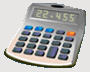DosageHelp.com Helping Nursing Students Prepare for Medication Exams By Explaining Dosage Calculations

# Volume/Time - IV Drop Rate Questions

Given a certain amount of liquid, a time period, and a drop factor (gtts/mL), what is the necessary IV flow rate in gtts/min? Measurement used when IV is regulated manually. Because it is not possible to give a patient a fraction of a drop, it is typical to round answers for these problems up or down to the nearest whole number.

Formula:
 Volume (mL) Time (min)
x Drop Factor (gtts/mL)  =  Y (Flow Rate in gtts/min)

Example: Calculate the IV flow rate for 1200 mL of NS to be infused in 6 hours. The infusion set is calibrated for a drop factor of 15 gtts/mL.
 Volume (mL) Time (min)
x Drop Factor (gtts/mL)  =  Y (Flow Rate in gtts/min)
Convert 6 hours to minutes.
• min ← hr    ( x by 60 )
• 6 hr x 60 = 360 min
 1200 mL 360 min
x 15 gtts/mL  =  50 gtts/min

Example: Calculate the IV flow rate for 200 mL of 0.9% NaCl IV over 120 minutes. Infusion set has drop factor of 20 gtts/mL.
 Volume (mL) Time (min)
x Drop Factor (gtts/mL)  =  Y (Flow Rate in gtts/min)
 200 mL 120 min
x 20 gtts/mL  =  33 gtts/min

Home Dosage Question Steps Unit Conversion Mass for Mass Mass/Liquid For Liquid IV Terms & Abbreviations Amount in IV Fluid Volume/Time - IV mL Rate Volume/Time - IV Drop Rate Fluid Maintenance Dosage By Weight Mass/Time - IV mL Rate Practice Questions Question of the Day Tell a Friend Print a Flyer